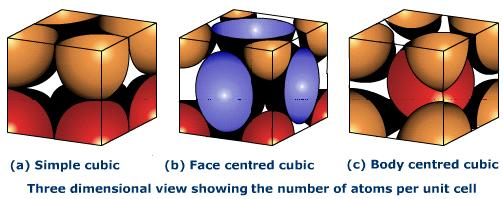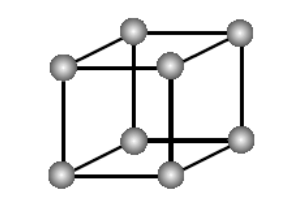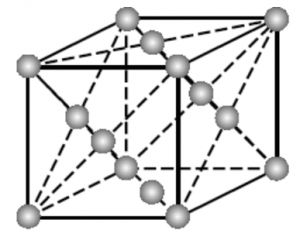# Number of Atoms in a Unit CellNumber of Atoms in a Unit Cell

This topic briefly explains about the number of atoms in a unit cell and the types of unit cell. Large number of unit cells together make a crystal lattice. Constituent particles like atoms, molecules are also present. Each lattice point is occupied by one such particle.

## Types of unit cell:

• ### Primitive cubic unit cell

1. The atoms in primitive cubic unit cell are present only at the corners
2. Every atom at the corner is shared among eight adjacent unit cells
3. Four unit cells are present in the same layer
4. Four unit cell in the upper/lower layer
5. Therefore a particular unit cell has only ${\frac{1}{8}}^{th}$ of an atom
6. Each small sphere in the following figure represents the center of a particle which occupies that particular position and not its size
7. Below is an open structureEach cubic unit cell consists 8 atoms at the corners, hence total number of atoms in one unit cell is: $8 ~×~\frac{1}{8}$ = $1$ atom

• ### Body centered cubic unit cell

1. In BCC unit cell every corner has atoms
2. There is one atom present at the center of the structure
3. Below diagram is an open structure
4. According to this structure atom at the body centers wholly belongs to the unit cell in which it is presentImage alt tag: bcc structure

Number of atoms in BCC cell:

1. a) $8$ corners × $\frac{1}{8}$ per corner atom = $8~ ×~ \frac{1}{8}$ = $1$ atom
2. b) $1$ body center atom = $1 ~× ~1$ = $1$ atom

So, total number of atoms present per unit cell = 2 atoms.

• ### Face centered cubic unit cell

1. In FCC unit cell atoms are present in all the corners of the crystal lattice
2. Also there is an atom present at the center of every face of the cube
3. This face-center atom is shared between two adjacent unit cells
4. Only 12 of each atom belongs to a unit cell
5. The diagram shown below is an open structureNumber of atoms in BCC cell:

1. a) $8$ corners × $\frac{1}{8}$ per corner atom = $8$ × $\frac{1}{8}$ = $1$ atom
2. b) $6$ face-centered atoms × $\frac{1}{2}$ atom per unit cell = $3$ atoms

Hence the total number of atoms in a unit cell = 4 atoms

This article explains the number of atoms in a unit cell of a crystal lattice. For any further query install Byju’s -the learning app.